# LDO Linear Regulators Paralleling with MAX38908 for High Current Applications

### Abstract

For high load current applications, it sometimes becomes very difficult to find a single LDO linear regulator to meet the load requirements due to current rating of the LDO or package limitation. To achieve such higher load current demands, paralleling the LDO’s will be one option to share the load current and the heat dissipation. This application note discusses different methods of LDO paralleling and compares the load sharing performance.

#### Introduction

As the demand of high performance and high power portable electronic devices are growing, the demands for high current LDO's with lower noise levels are increasing. It is becoming difficult for a single LDO to handle the higher loads due to the current limit and limitation of the junction temperature. The heat dissipated in the LDO also increases when input to output voltage difference is more with high current requirements. In these situations, paralleling the LDO's is the best option to handle the required load demands and heat distribution. This application note discusses about the different ways to parallel the LDO's with MAX38908 high performance LDO's and compares the load sharing performances.

#### About MAX38907/8/9 4A/2A High-Performance LDO Linear Regulators:

The MAX38907/MAX38908/MAX38909 are fast transient response, high PSRR NMOS LDO's input supply ranges from 0.9V up to 5.5V, and BIAS voltage range from 2.7V to 20V. This family of LDO's can deliver up to 4A Load Current at 82mV Dropout. The output voltage on the MAX38908/MAX38909 can be adjusted to a value in the range of 0.6V to 5.0V by using two external feedback resistors.

Features Performance
• Reduces Noise and Improves Accuracy
• 28mV 4A Load Transient Excursion
• 78dB BIAS PSRR at 10kHz
• 52dB IN PSRR at 10kHz
• Enables Ease-of-Use and Robust Protection
• Programmable Soft-Start Rate
• Overcurrent and Overtemperature Protection
• Output-to-Input Reverse Current Protection
• Power-OK Status Pin
• Reduces Size, Improves Reliability
• 14-pin (3mm x 3mm) TDFN
• 20-pin (5mm x 5mm) TQFN, and 5 x 3 Bump, 0.4mm Pitch WLP PackagesFigure 1. VIN PSRR graph.

The application circuit of the MAX38908 LDO is given below Figure 2.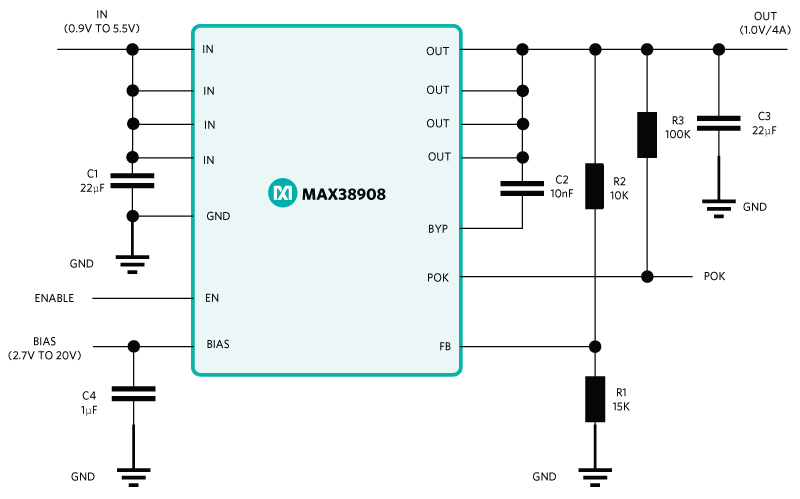Figure 2. MAX38908 Application circuit

#### Paralleling of LDO's:

Basically, we will study about the different ways of paralleling of the LDO's and we will compare the load sharing results. The following are the different topologies of paralleling the LDO's.

1. LDO direct paralleling.
2. LDO paralleling using balancing resistors.
3. LDO paralleling using op-amp.

For this study we are considering the following use case:

VIN = 3.3V, VOUT = 2.7V, IOUT = 7A, VBIAS = 10V

1. LDO direct paralleling: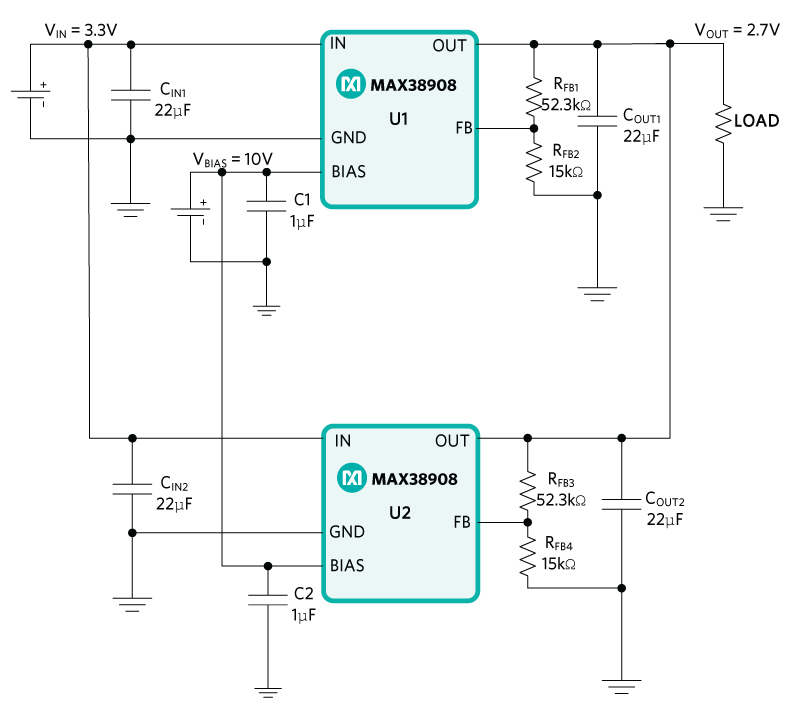Figure 3. MAX38908 LDO's paralleled directlyFigure 4. Load sharing in direct LDO paralleled circuit

From above Figure 4, the LDO-U2 which has slightly more output voltage among both is supplying the load current till 4Amp. If the load is further increased beyond the maximum allowable load current of the LDO, the LDO-U2's output voltage will reduce below the set value and LDO-U1 will share the current. Once the current starts flowing from LDO-U1, the current from LDO-U2 reduces and the output voltage reaches the set value, it again starts sharing more current. Hence, the maximum load will be shared by LDO-U2 due to the unequal output voltage.

2. Paralleling of LDO's with balancing resistors:

The following Figure 5 shows parallel connection of the LDO's using the balancing resistors at the output. As shown in the Figure 5, the IN pins of the two LDO's are shorted and connected directly to input voltage source but the OUT pins are connected to load through the balancing resistors which are of same value. In this circuit, the LDO which has higher output voltage supplies current and this current creates voltage drop across that balancing resistor, hence reduces the actual output voltage of that LDO. Thus, the other LDO with lower output voltage will also share the load current. Hence, the drop-in voltage across the balancing resistor will help to balance the output voltage while balancing the current flowing through both the LDO's.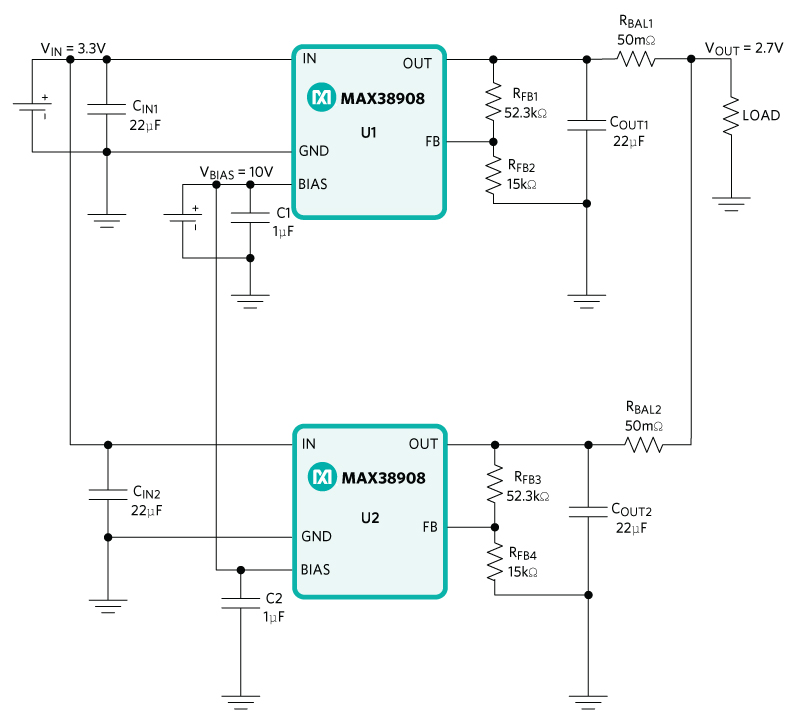Figure 5: MAX38908 LDO's paralleled with balancing resistor

The voltage across the load with balancing resistors is given as:

VLOAD = VOUTU1 - VRBAL1 = VOUTU2 - VRBAL2
VLOAD = VOUTU1 - (IOUTU1*RBAL1) = VOUTU2 - (IOUTU2 * RBAL2)

To calculate the balancing resistor value, we consider the accuracy of the output voltage of LDO's is ±1% and allowed difference in maximum sharing of output current from each LDO is considered as 20% of maximum output of each LDO i.e., 800mA. Following equation gives the value of balancing resistor.As the output voltage is 2.7V, the output voltage variation will be 54mV. then the balancing resistance is calculated to be 67.5m?. So, the selected balancing resistance is 50m?.Figure 6. Load sharing in LDO parallel circuit using balancing resistor

From equation Rbal, the load current sharing can be still be improved by increasing the balancing resistor. By increasing the balancing resistor value further, the voltage drop across balancing resistor will further increase and actual output voltage will reduce. We need to make sure that the balance resistor value should be chosen such that the actual output voltage across the load will not reduce significantly. So, choose the balance resistor value such that the current difference through LDO's is less and the voltage drop across the balancing resistor is also less. Good layout design will help in equally sharing the current through the LDO's.

3. Paralleling of LDO's using op-amp:

In the below circuit of Figure 7, the parallel connection of the LDO's is done through the input sense resistors. There are two resistors connected from input of the source and IN terminals of the two LDO's. Once the current flows through each resistors the voltage is developed proportionally across them and these voltages are fed to an op-amp as shown in the Figure. The op-amp senses the differential voltage given to its two input terminals and it drives the feedback of the LDO-U1 which has its current sense resistor connected to the inverting pin. From the following Figure, if the current from RSENSE2 increases, the op-amp will sense the increase in current and starts to sink the current from feedback network of LDO-U1. This current sink causes the LDO-U1 to take more current from input source and thus increasing the drop on RSENSE1 resistor. Hence it acts as the negative feedback to balance the current flowing through the both LDO's. When the circuit operating in the steady state condition, the current and output voltages from U1, U2 will be balanced.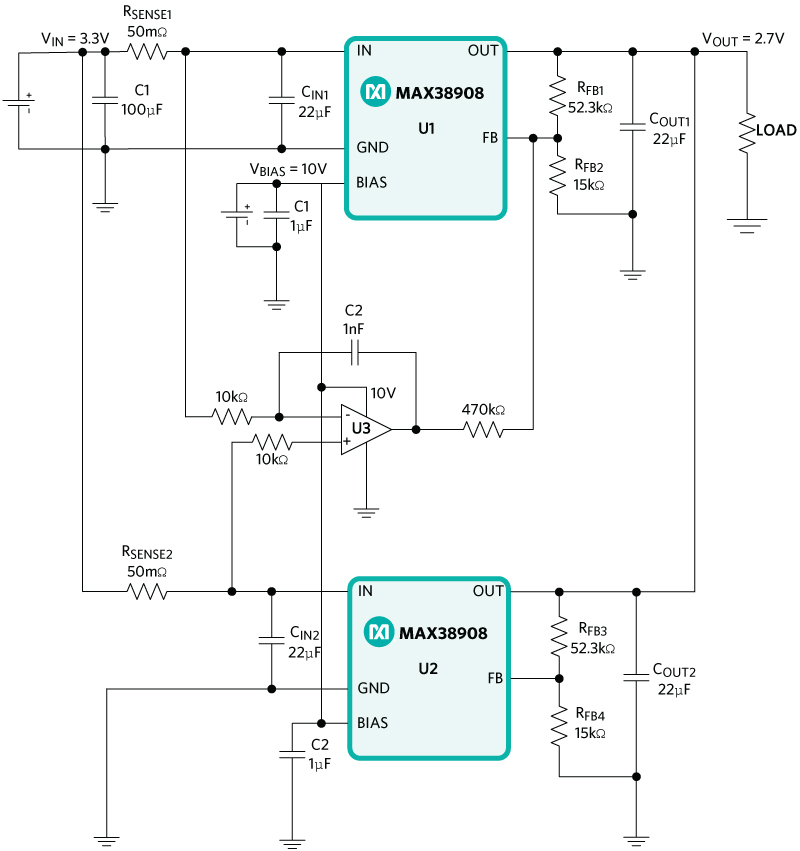Figure-7: MAX38908 LDO's paralleled using op-amp

In the above circuit, we have used 50m? current sense resistors at each LDO input for current sensing and an op-amp is used to compare the input current flowing through two LDO's. We can see that the current sharing through the LDO's is much better than the earlier methods and the current sharing graph using this method is given below. The maximum difference in the currents flowing through both the LDO's is around 51mA throughout the load current range till 7Amp.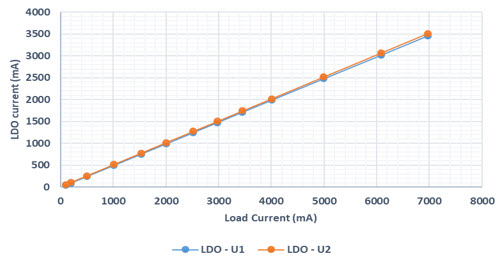Figure 8. Load sharing in LDO parallel circuit using Op-amp

Start-up performance:Figure 9. Start-up performance at test condition: VIN = 3.3V, VOUT = 2.7V, IOUT = 7.3Amp

In the above waveform, Green - Input voltage, Pink - Output voltage, Yellow - LDO-U1 current, Blue - LDO-U2 current.

The above Figure 9 shows the start-up waveform where we can see how the current balancing works. During start-up, the LDO-U2 takes more current which is sensed by op-amp and immediately balances the current through both LDO's. The currents flowing through both the LDO's are given in yellow and blue waveforms, which are settled in sometime and becomes equal.

Below are the waveforms of load transient and load regulation data of LDO parallel circuit using op-amp.Figure 10. Load transient performance at test condition: VIN = 3.3V, VOUT = 2.7V, IOUT = 0 to 7.3Amp

Output voltage drop while load transient from 0 to 7.3A - 82mVFigure 11. Load regulation performance at test condition: VIN = 3.3V, VOUT = 2.7V, IOUT = 0 to 7Amp

#### Performance comparison of LDO paralleling techniques:Figure 12. Load transient at test condition: VIN = 3.3V, VOUT = 2.7V, IOUT = 0 to 7Amp

The above waveform shows the load regulation performance of both the circuits of Figure 5 & 7. As the load increases, the output voltage of the circuit using balancing resistors reduces much more than of the op-amp circuit.Figure 13. Load transient at test condition: VIN = 3.3V, VOUT = 2.7V, IOUT = 0 to 7Amp